Courses

# Propositional And First Order Logic MCQ - 2

## 20 Questions MCQ Test RRB JE for Computer Science Engineering | Propositional And First Order Logic MCQ - 2

Description
This mock test of Propositional And First Order Logic MCQ - 2 for Computer Science Engineering (CSE) helps you for every Computer Science Engineering (CSE) entrance exam. This contains 20 Multiple Choice Questions for Computer Science Engineering (CSE) Propositional And First Order Logic MCQ - 2 (mcq) to study with solutions a complete question bank. The solved questions answers in this Propositional And First Order Logic MCQ - 2 quiz give you a good mix of easy questions and tough questions. Computer Science Engineering (CSE) students definitely take this Propositional And First Order Logic MCQ - 2 exercise for a better result in the exam. You can find other Propositional And First Order Logic MCQ - 2 extra questions, long questions & short questions for Computer Science Engineering (CSE) on EduRev as well by searching above.
QUESTION: 1

### Identify the correct translation into logical notation of the following assertion. "Some boys in the class are taller than all the girls" Note : taller(x,y) is true if x is taller than y.

Solution:

Now many people get confused when to use ∧ and when to use →. This question tests exactly that. We use ∧ when we want to say that the both predicates in this statement are always true, no matter what the value of x is. We use → when we want to say that although there is no need for left predicate to be true always, but whenever it becomes true, right predicate must also be true. D means there exist some boys x which taller than all girls y.

QUESTION: 2

### The following propositional statement is (P → (Q v R)) → ((P ^ Q) → R)

Solution:

We create the truth table for given statement S as :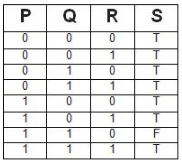A formula is satisfiable if there is atleast one assignment for which it is true. Clearly this formula is satisfiable as there are 7 assignments for which it is true. A formula is valid if it is true for all assignments, which is not the case here. Thus, option (A) is correct. Please comment below if you find anything wrong in the above post.

QUESTION: 3

### Which of the following is a valid first order formula ? (Here α and β are first order formulae with x as their only free variable)

Solution:
QUESTION: 4

Consider the following formula a and its two interpretations I1 and I2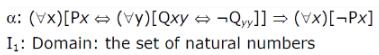Px = 'x is a prime number '

Qxy = ' y divides x'

I2 : same as I1 except that Px - 'x is a composite number'

Q.
Which of the following statements is true?

Solution:

First of all, note that, in α, ¬Qyy is always false, because every number divides itself. Also not that rightmost formula (∀x)[¬Px] is always false, because clearly it is not the case that every number is not the prime number (in case of I1), nor it is the case that every number is not the composite number (in case of I2). Also note that, variable x in this expression is not same as variable x in leftside expression, they are independent. In fact, we can rewrite α as α:(∀x)[Px⇔(∀y)[Qxy⇔¬Qyy]]⇒(∀z)[¬Pz]. Let us consider I1 first. So let us assign some value to x, and see if it satisfies α. We can partition assignments of x into 3 parts : when x is prime, when x is composite, when x is 1.

• When x is prime : Px is true, also Qxy is false for all y except 1, because only 1 divides x. So formula Qxy⇔¬Qyy is true for all y except 1, but due to ∀y outside this, whole formula ∀y[Qxy⇔¬Qyy] becomes false, because it would have been true if Qxy⇔¬Qyy was true for every y. So now [Px⇔(∀y)[Qxy⇔¬Qyy]] becomes false for all x whenever x is prime. Since for some x (where x is prime), [Px⇔(∀y)[Qxy⇔¬Qyy]] is false, so (∀x)[Px⇔(∀y)[Qxy⇔¬Qyy]] is definitely false, since false⇒ false is true, so α is true in I1, and we don't need other cases of x.
• Now consider I2. Here also we can argue in the same way as we did in cases of I1, here case of x being composite leads to false⇒false, and so α is also true in I2, hence option (D) is correct.
QUESTION: 5

Consider the following logic program P A(x) <- B(x, y), C(y) <- B(x,x) Which of the following first order sentences is equivalent to P?

Solution:
QUESTION: 6

The following resolution rule is used in logic programming:

Derive clause (P v Q) from clauses (P v R), (Q v ¬ R)

Q.
Which of the following statements related to this rule is FALSE?

Solution:
QUESTION: 7

"If X, then Y unless Z" is represented by which of the following formulae in propositional logic? ("¬" is negation "^" is conjunction, and "→" is implication)

Solution:

The statement "If X then Y unless Z" means, if Z doesn't occur, X implies Y i.e. ¬Z→(X→Y), which is equivalent to Z∨(X→Y) (since P→Q ≡ ¬P∨Q), which is then equivalent to Z∨(¬X∨Y). Now we can look into options which one matches with this.
So option (a) is (X∧¬Z)→Y = ¬((X∧¬Z))∨Y = (¬X∨Z)∨Y, which matches our expression. So option A is correct.

QUESTION: 8

Consider two well-formed formulas in prepositional logic.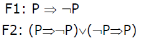Q.
Which of the following statements is correct?

Solution:

The concept behind this solution is: a) Satisfiable If there is an assignment of truth values which makes that expression true. b) UnSatisfiable If there is no such assignment which makes the expression true c) Valid If the expression is Tautology Here, P => Q is nothing but –P v Q F1: P => -P = -P v –P = -P F1 will be true if P is false and F1 will be false when P is true so F1 is Satisfiable F2: (P => -P) v (-P => P) which is equals to (-P v-P) v (-(-P) v P) = (-P) v (P) = Tautology So, F1 is Satisfiable and F2 is valid Option (a) is correct.

QUESTION: 9

Let a, b, c, d be propositions. Assume that the equivalences a ↔ (b V-b) and b ↔ c hold. Then the truth value of the formula (a ∧ b) → (a ∧ c) ∨ d) is always

Solution: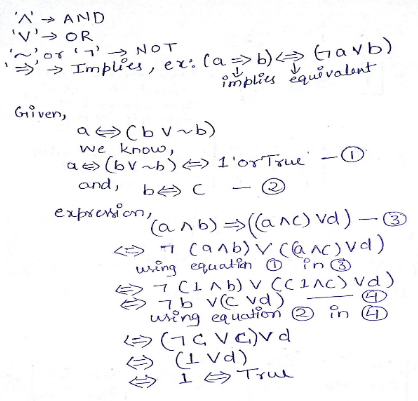QUESTION: 10

Which one of the following is not equivalent to p←→q

Solution:

This question is the revision of basics of propositional logic. Conjunction Conjunction of p and q, denoted by p ∨q, is the proposition ‘p or q’.The Conjunction p ∨q is when anyone of p or q is TRUE. Disjunction Disjunction of p and q, denoted by p ∧q, is the proposition ‘p and q’. The Disjunction p ∧q is TRUE when both p and q is TRUE. Logical Implication It is a type of relationship between two statements or sentence. Denoted by ‘p → q’. The conditional statement p → q is false when p is true and q is false, and true otherwise. i.e. p→ q = ¬p ∨q Bi-Condition A bi-conditional statement is a compound statement formed by combining two conditionals under “and.” Bi-conditionals are true when both statements have the exact same truth value. Solution : p←→q means both p→q and q→p p→q is equivalent to ⌉p ∨ q So A and B are fine. D is a different way of writing A p ↔ q = (p→ q) ∧ (q→p) (⌉p ∨ q) ∧ (q → p) ( As p→ q = ⌉p ∨ q) (⌉p ∨ q) ∧ (⌉q ∨ p) = (¬p ∧ p)∨ (¬p ∧¬q)∨ (q ∧p) ∨(q ∧¬q) (Distributive law) As ((¬p∧ p)=0,(q ∧¬q)=0) (Complementation) (⌉p ∧ ⌉q) ∨ (p ∧ q) So, answer C

QUESTION: 11

Consider the following two statements.

S1: If a candidate is known to be corrupt, then he will not be elected.
S2: If a candidate is kind, he will be elected.

Q.
Which one of the following statements follows from S1 and S2 as per sound inference rules of logic?

Solution:

S1: If a candidate is known to be corrupt, then he will not be elected.
S2: If a candidate is kind, he will be elected. If p → q, then ¬q → ¬p
So from S1, elected → not corrupt
and S2 is, kind → elected
Therefore, kind → not corrupt

QUESTION: 12

Which one of the following well formed formulae is a tautology?

Solution:

In logic, a tautology is a formula that is true in every possible interpretation. All options here are based on order of application of quantifier. So, there are 2 rules:

• The positions of the same type of quantifiers can be switched
• The positions of different types of quantifiers cannot be switched.

Now, let's see the Choices of the question: Option (a) Sign <-> represents "not equivalent".

∀x∃y R( x, y) is not equivalent to ∃Y ∀X RX, Y)

Let R(X, Y) represent X < Y for the set of numbers as the universe, for example. Then ∀X ∃Y R(X, Y) reads "for every number x, there is a number y that is greater than x", which is true, while ∃Y ∀X R(X, Y) reads "there is a number that is greater than every (any) number", which is not true. So this option is rejected. Option (d) Sign -> represents "euivalent"

∀X ∀Y R(X, Y) is equivalent to ∀X ∀Y R(Y, X)

Let R(X, Y) represent X < Y for the set of numbers as the universe, for example. Then ∀X ∀Y R(X, Y) reads "for every number X, there is every Y that is greater than x", while ∀X ∀Y R(Y, X) reads "for every number Y, there is every X that is greater than Y". And both can’t be equivalent (because at one time, one will be true and other one will be false) So this option is rejected. Option (b) is clearly rejected as two predicate can’t be equivalent to one predicate only. So Option (c) is the correct one. Explanation for Pption (c) – as position of the quantifier is not changed and since LHS P -> R = ⌐P ᴠ R which is equal to RHS. Option c is tautology and correct answer too. Note: For solving proposition logic question, always remember not to try with rules only. Just take an example and see if options are satisfying it or not. Because for a particular example, three options will give same result and one will be different. And different one is your answer. For basics to this question, you can refer to:

QUESTION: 13

Let # be a binary operator defined as X # Y = X′ + Y′ where X and Y are Boolean variables. Consider the following two statements.

S1: (P # Q) # R = P # (Q # R)
S2: Q # R = R # Q

Q.
Which of the following is/are true for the Boolean variables P, Q and R?

Solution:

S2 is true, as X' + Y' = Y' + X'

S1 is false.
Let P = 1, Q = 1, R = 0, we get different results
(P # Q) # R = (P' + Q')' + R' = (0 + 0)' + 1 = 1 + 1 = 1
P # (Q # R) = P' + (Q' + R')' = 0 + (0 + 1)' = 0 + 0 = 0

QUESTION: 14

Suppose U is the power set of the set S = {1,2,3,4,5,6}. For any T ∈ U, let |T| denote the number of elements in T and T′ denote the complement of T. For any T, R ∈ U, let TR be the set of all elements in T which are not in R. Which one of the following is true?

Solution:

D is true, it can be seen by drawing Venn Diagram.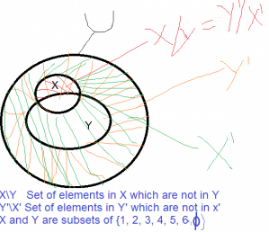A is false, Take an example like X = {1, 2, 3, 4},
X' = {5, 6}, |X| is not same as |X'|.

B is false, as any two subsets of size 5 of U would definitely have some common elements.

C is false, Take an example like X = {1, 2} Y = {3, 4, 5}, XY = {1, 2}.

QUESTION: 15

In a room there are only two types of people, namely Type 1 and Type 2. Type 1 people always tell the truth and Type 2 people always lie. You give a fair coin to a person in that room, without knowing which type he is from and tell him to toss it and hide the result from you till you ask for it. Upon asking, the person replies the following:

“The result of the toss is head if and only if I am telling the truth.”

Q.
Which of the following options is correct?

Solution:

“The result of the toss is head if and only if I am telling the truth.”

If the person is of Type 1 who always tell truth, then result must be head.

If the person is of Type 2 who always tell lie, then result must be head.

Negation of a sentence of the form "X is true if and only if Y is true" is "Either X is true and Y is false, or X is false and Y is true."

Which means "Either toss is head and I am not telling truth, or toss is tail and I am telling truth".

Since the person always lie, it is "Either toss is head and I am not telling truth"

QUESTION: 16

Let p,q,r and s be four primitive statements. Consider the following arguments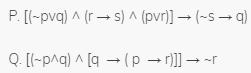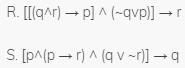Q.

Which of the above arguments are valid?

Solution:
QUESTION: 17

Let P(x) and Q(x) be arbitrary predicates. Which of the following statements is always TRUE?

Solution:

Generally, these type of questions can be solved by using two statements and checking the validity of each and every option. Here, let the statements be P and Q where, P : Student is a girl Q : Student is smart Option B says that, IF for all student x : If x is a girl then the student is smart THEN IF the whole class comprises of girls then the whole class comprises of smart students.

QUESTION: 18

Consider the following expressions:
(i) false    (ii) Q
(iii) true (iv) P ∨ Q
(v) ¬Q ∨ P
The number of expressions given above that are logically implied by P ∧ (P ⇒ Q) is ______________

[This Question was originally a Fill-in-the-blanks Question]

Solution: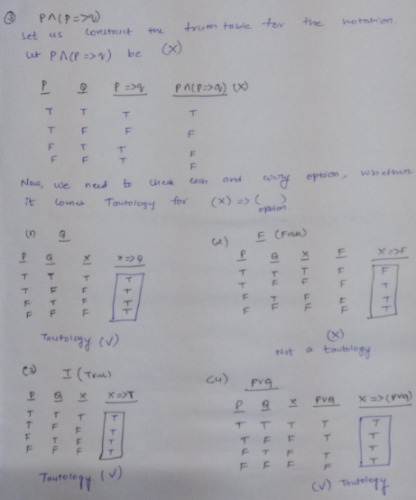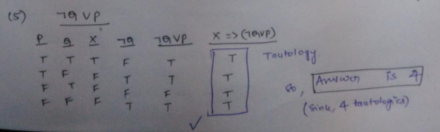This solution is contributed by Anil Saikrishna Devarasetty.   Alternate Explanation : Answer is 4. Here is the solution If say X is 'Logically Implied' by [P ∧ (P ⇒ Q)] then [P ∧ (P ⇒ Q)] ⇒ X is always true i.e it is a tautology so if the above expression is a tautology then we can say that X is logically implied by P ∧ (P ⇒ Q) So we need to find X for which [P ∧ (P ⇒ Q)] ⇒ X will be always true for all values of P, Q and X. Look at the below table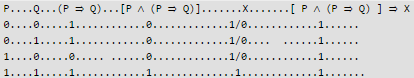notice that value of X doesn't matter if premise of expression i.e Premise of [P ∧ (P ⇒ Q)] ⇒ X i.e [P ∧ (P ⇒ Q)] is 0 meaning the final expression would be a tautology for all values of X if [P ∧ (P ⇒ Q)] is 0 but if premise is 1 (as in last row) then X must be 1 so that the final implication i.e., [P ∧ (P ⇒ Q)] ⇒ X is true for all values. if you replace X by all 5 options then you will find that for X = Q, True, P ∨ Q, ¬Q ∨ P the said expression would always be true for X = False the expression would not be a tautology Hence # of expression is 4 ------------------------------------------------------------------

Note: An important inference rule called "modus ponenes" says this [P ∧ (P ⇒ Q)] ⇒ Q is a tautology we noted that if we replace X by Q then it is indeed a tautology meaning Q is implied by [P ∧ (P ⇒ Q)]

QUESTION: 19

Which one of the following well-formed formulae in predicate calculus is NOT valid?

Solution:

Suppose if there are two statements P and Q, P=>Q = ~PvQ i.e.

The only situation where implication fails is (=>) when P is true and Q is false. i.e. A truth statement can't imply a false statement.

So, for these type of questions it will be better to take option and check for some arbitrary condition

By looking options, we are pretty sure that A,B are correct

Suppose X is any number and statement is P(x) = X is a prime number
Q(x) = X is a non-prime number
If we look at option D,

Before Implication :- For all x, x is either prime or non-prime which is true
After Implication :- For all x, x is prime or for all x, x is non-prime which is obviously false i.e. here, truth statement implies a false statement which is not valid.

If we carefully look at option C, There exists a number x, which is both prime and non-prime which is false and a false statement can imply either true or false. So option (C) is correct So Answer is Option (D)

QUESTION: 20

Which one of these first-order logic formula is valid?

Solution:

(A) LHS->RHS
LHS: For every x (if P holds then Q holds)
RHS: If P(x) holds for all x, then Q(x) holds for all x.
(B) LHS !->RHS
LHS: An x exist for which either P(x) is true or Q(x) is true.
RHS: If an x exist for which P(x) is true then another x exist for which Q(x) is true.
(C) It is not necessary that on RHS both x are same.
LHS: There exist an x for which both P(x) and Q(x) are true.
RHS: There exist an x for which P(x) is true and there exist an x for which Q(x) is true.
(D) LHS!->RHS
LHS: For every x, there exist a y such that P(x, y) holds.
RHS: There exist a y such that for all x P(x, y) holds.